# 基于像增强器的紫外光谱探测系统中的噪声分析Noise Analysis in UV Spectral Detection Systems Based on Image Intensifiers

DOI: 10.12677/APP.2021.111011, PDF, HTML, XML, 下载: 141  浏览: 891

Abstract: In order to substantially improve the sensitivity of UV spectral detection, this paper couples the UV ICCD, which is made by coupling the UV image intensifier and CCD device, with the spectral component again to form a direct reading UV spectral detection system. The UV spectral detection system based on the image intensifier is designed, and the noise of the UV image intensifier and the noise of the CCD camera are specifically analyzed respectively. The experimental results of the study show that the longer the exposure time is, the less noise the CCD image has and the smoother the grey scale curve is; conversely, the shorter the exposure time is, the more the CCD image is affected by noise and the more drastic the grey scale curve is. It is found that the extension of the frame integration time can effectively reduce the random noise and make the image signal-to-noise ratio higher. The work in this paper provides a basis for reducing the impact of noise on the system and thus improving the detection efficiency, greatly expanding the application area of UV spectral detection technology, and providing a basis for the development of micro-optical night vision technology.

1. 引言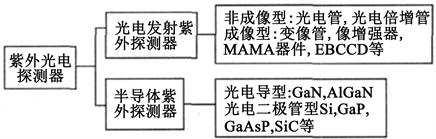Figure 1. Classification of UV detectors

2. ICCD的结构与工作原理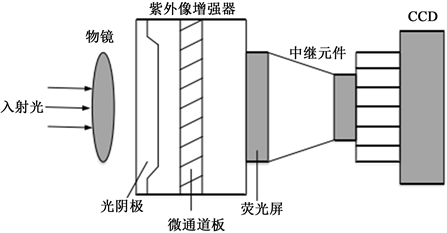Figure 2. Schematic diagram of UV ICD structure

CCD与像增强器之间的耦合中继元件有两类：1) 光纤光锥：光纤光锥的结构为一头大、一头小，它是一种光纤传像器件。2) 中继透镜：这种方式也可耦合像增强器的输出图像到CCD的输入光敏面上，而且容易调节，获得的图像也很清楚，因此，这种耦合的方式对于背照明和前照明的CCD都很适合。

3. 紫外ICCD的噪声模型

3.1. 图像噪声的基本理论模型

$n\left(r,t\right)=\left[\begin{array}{c}{n}_{r}\left(t\right)\\ {n}_{t}\left(r\right)\end{array}\right]$ (1)

$\Phi =\epsilon \left[n\left(r,t\right){n}^{\text{T}}\left(r+\rho ,t+\tau \right)\right]$ (2)

$\Phi =\left[\begin{array}{cc}\epsilon \left[{n}_{r}\left(t\right){n}_{r+\rho }\left(t+\tau \right)\right]& \epsilon \left[{n}_{r}\left(t\right){n}_{t+\tau }\left(r+\rho \right)\right]\\ \epsilon \left[{n}_{t}\left(r\right){n}_{r+\rho }\left(t+\tau \right)\right]& \epsilon \left[{n}_{t}\left(r\right){n}_{t+\tau }\left(r+\rho \right)\right]\end{array}\right]$ (3)

$\Phi =\left[\begin{array}{cc}{\Phi }_{r,r+\rho }\left(t,t+\tau \right)& {\Phi }_{r,t+\tau }\left(t,r+\rho \right)\\ {\Phi }_{t,r+\rho }\left(r,t+\tau \right)& {\Phi }_{t,t+\tau }\left(t,r+\rho \right)\end{array}\right]$ (4)

${\Phi }_{r,t+\tau }\left(t,r+\rho \right)={\Phi }_{t,r+\rho }\left(r,t+\tau \right)=0$ (5)

$\Phi =\left[\begin{array}{cc}{\Phi }_{r,r+\rho }\left(t,t+\tau \right)& 0\\ 0& {\Phi }_{r,r+\tau }\left(r,r+\rho \right)\end{array}\right]$ (6)

${\Phi }_{r,r+\rho }\left(t,t+\tau \right)={\Phi }_{r,r}\left(t,t+\tau \right)={\Phi }_{1}\left(t,t+\tau \right)$ (7)

${\Phi }_{r,r+\tau }\left(r,r+\rho \right)={\Phi }_{r,r}\left(r,r+\rho \right)={\Phi }_{2}\left(r,r+\rho \right)$ (8)

$\Phi =\left[\begin{array}{cc}{\Phi }_{1}\left(t,t+\tau \right)& 0\\ 0& {\Phi }_{2}\left(r,r+\rho \right)\end{array}\right]$ (9)

${\Phi }_{1}\left(t,t+\tau \right)={\Phi }_{1}\left(\tau \right)$ (10)

${\Phi }_{2}\left(r,r+\rho \right)={\Phi }_{2}\left(\rho \right)$ (11)

$\Phi =\left[\begin{array}{cc}{\Phi }_{1}\left(\tau \right)& 0\\ 0& {\Phi }_{2}\left(\rho \right)\end{array}\right]$ (12)

$W\left(v,f\right)=\left[\begin{array}{cc}{W}_{1}\left(v\right)\delta \left(f\right)& 0\\ 0& {W}_{2}\left(f\right)\delta \left(v\right)\end{array}\right]$ (13)

${W}_{1}\left(v\right)={\int }_{-\infty }^{\infty }{\Phi }_{1}\left(\tau \right){\text{e}}^{-2\pi jv\tau }\text{d}\tau$ (14)

${W}_{2}\left(f\right)={\int }_{-\infty }^{\infty }{\Phi }_{2}\left(\rho \right){\text{e}}^{-2\pi jf\rho }\text{d}\rho$ (15)

$V=\left[\begin{array}{cc}{\int }_{-\infty }^{\infty }{W}_{1}\left(v\right)\text{d}v& 0\\ 0& {\int }_{-\infty }^{\infty }{W}_{2}\left(f\right)\text{d}f\end{array}\right]$ (16)

3.2. 系统噪声

$\beta ={\cap }_{i=1}^{M}{\beta }_{i}$ (17)

${\beta }_{im}={\cap }_{j=i+1}^{m}{\beta }_{j}$ (18)

$H\left(f\right)={\cap }_{i=1}^{M}{H}_{i}\left({\beta }_{im}f\right)$ (19)

$M\left(f\right)={\cap }_{i=1}^{M}{M}_{j}\left({\beta }_{im}f\right)$ (20)

${f}_{m}$ 为第m级子系统输出端的空间频率

${f}_{m}={\beta }_{mM}f$ (21)

${H}_{im}\left({f}_{m}\right)={\cap }_{j=i+1}^{m}{H}_{i}\left({\beta }_{jm}{f}_{m}\right)$ (22)

${M}_{im}\left({f}_{m}\right)={\cap }_{j=i+1}^{m}{M}_{j}\left({\beta }_{jm}{f}_{m}\right)$ (23)

${\alpha }_{i}=\frac{{\int }_{0}^{\infty }{p}_{i}\left(\lambda \right){\epsilon }_{i}\left(\lambda \right)\text{d}\lambda }{{\int }_{0}^{\infty }{p}_{i}\left(\lambda \right)\text{d}\lambda }$ (24)

$G={\cap }_{i=1}^{M}{a}_{i}{g}_{i}$ (25)

${G}_{im}={\cap }_{j=i+1}^{m}{a}_{j}{g}_{j}$ (26)

${W}_{2}\left(f\right)={\sum }_{i=0}^{M}{W}_{2i}\left({\beta }_{iM}f\right){G}_{iM}^{2}{M}_{IM}^{2}\left(f\right)$ (27)

${W}_{2ic}\left({f}_{i}\right)={\sum }_{j=0}^{i}{W}_{2i}\left({\beta }_{ji}{f}_{i}\right){G}_{ji}^{2}{M}_{ji}^{2}\left({f}_{i}\right)$ (28)

${W}_{1}\left(v\right)={\sum }_{i=0}^{M}{W}_{1i}\left(v\right){G}_{iM}^{2}{M}_{iM}^{2}\left(v\right)$ (29)

${M}_{iM}\left(v\right)={\cap }_{j=i+1}^{m}{M}_{j}\left(v\right)$ (30)

${M}_{j}\left(v\right)$ 为第j级子系统的时间域调制传递函数。且有类似约定： ${G}_{MM}={M}_{MM}\left(v\right)=1$。包括为第级在内的第 级以前系统累积时间噪声的维纳谱则为

${W}_{1ic}\left(v\right)={\sum }_{j=0}^{i}{W}_{1j}\left(v\right){G}_{ji}^{2}{M}_{ji}^{2}\left(v\right)$ (31)

${M}_{ji}\left(v\right)={\cap }_{q=j+1}^{i}{M}_{q}\left(v\right)$ (32)

${\sigma }_{2}^{2}={\int }_{-\infty }^{\infty }{W}_{2}\left(f\right)\text{d}f$ (33)

${\sigma }_{1}^{2}={\int }_{-\infty }^{\infty }{W}_{1}\left(v\right)\text{d}f$ (34)

${\sigma }_{2ic}^{2}={\int }_{-\infty }^{\infty }{W}_{2ic}\left({f}_{i}\right)\text{d}{f}_{i}$ (35)

${\sigma }_{1ic}^{2}={\int }_{-\infty }^{\infty }{W}_{1ic}\left(v\right)\text{d}v$ (36)

${\sigma }^{2}={\sigma }_{2}^{2}+{\sigma }_{2}^{2}$ (37)

${\sigma }_{ic}^{2}={\sigma }_{2ic}^{2}+{\sigma }_{1ic}^{2}$ (38)

$F=\frac{\left(SNR\right){p}_{1in}}{\left(SNR\right){p}_{2out}}=\frac{{\sigma }_{sot}^{2}}{{\sigma }_{par}^{2}}$ (39)

${F}_{2i}=\frac{{\int }_{-\infty }^{\infty }{\sum }_{j=0}^{i}{W}_{2j}\left({\beta }_{ji}{f}_{i}\right){G}_{ji}^{2}{M}_{ji}^{2}\left({f}_{i}\right)\text{d}{f}_{i}}{{\int }_{-\infty }^{\infty }{\sum }_{j=0}^{i-1}{W}_{2j}\left({\beta }_{ji}{f}_{i}\right){G}_{ji}^{2}{M}_{ji}^{2}\left({f}_{i}\right)\text{d}{f}_{i}}=1+\frac{{\sigma }_{2i}^{2}}{{g}_{i{a}_{2}}^{2}{\sigma }_{2\left(i-1\right)c}^{2}}$ (40)

${\sigma }_{2i}^{2}={\int }_{-\infty }^{\infty }{W}_{2i}\left({f}_{i}\right)\text{d}{f}_{i}$ (41)

${g}_{i{\sigma }_{2}}^{2}=\frac{{\int }_{-\infty }^{\infty }{\sum }_{j=0}^{i-1}{W}_{2i}\left({\beta }_{ji}{f}_{i}\right){G}_{ji}^{2}{M}_{ji}^{2}\left({f}_{i}\right)\text{d}{f}_{i}}{{\int }_{-\infty }^{\infty }{\sum }_{j=0}^{i-1}{W}_{2j}\left({\beta }_{j\left(i-1\right)}{f}_{i-1}\right){G}_{j\left(i-1\right)}^{2}{M}_{j\left(i-1\right)}^{2}\left({f}_{i-1}\right)\text{d}{f}_{i-1}}$ (42)

M级级联系统对于空间噪声的总噪声因子为

${F}_{2}={\cap }_{i=1}^{M}{F}_{2i}$ (43)

${F}_{1i}=\frac{{\int }_{-\infty }^{\infty }{\sum }_{j=0}^{i}{W}_{1j}\left(v\right){G}_{ji}^{2}{M}_{ji}^{2}\left(v\right)\text{d}v}{{\int }_{-\infty }^{\infty }{\sum }_{j=0}^{i-1}{W}_{1j}\left(v\right){G}_{ji}^{2}{M}_{ji}^{2}\left(v\right)\text{d}v}=1+\frac{{\sigma }_{1i}^{2}}{{g}_{i{\sigma }_{1}}^{2}{\sigma }_{1\left(i-1\right)c}^{2}}$ (44)

${\sigma }_{1i}^{2}={\int }_{-\infty }^{\infty }{W}_{1i}\left(v\right)\text{d}v$ (45)

$i-1$ 级在内第 $i-1$ 级以前系统累积时间噪声的方差； ${g}_{i{\sigma }_{1}}^{2}$ 是第i级子系统输入时间噪声传递至第i级子

${g}_{i{\sigma }_{1}}^{2}=\frac{{\int }_{-\infty }^{\infty }{\sum }_{j=0}^{i-1}{W}_{1j}\left(v\right){G}_{ji}^{2}\left(v\right){M}_{ji}^{2}\left(v\right)\text{d}v}{{\int }_{-\infty }^{\infty }{\sum }_{j=0}^{i-1}{W}_{1j}\left(v\right){G}_{j\left(i-1\right)}^{2}{M}_{j\left(i-1\right)}^{2}\left(v\right)\text{d}v}$ (46)

M级级联系统对于时间噪声的总噪声因子为

${F}_{1}={\cap }_{i=1}^{M}{F}_{1i}$ (47)

${\sigma }_{1N}^{2}={\int }_{|l|\le \tau }{\int }_{r\in a}{\int }_{-\infty }^{\infty }{W}_{1n}\left(f\right)\text{d}f\text{d}r\text{d}t$ (48)

${\sigma }_{2N}^{2}={\int }_{|l|\le \tau }{\int }_{r\in a}{\int }_{-\infty }^{\infty }{W}_{2n}\left(f\right)\text{d}f\text{d}r\text{d}t$ (49)

4. 噪声分析与讨论

4.1. 光电阴极产生的噪声

${N}_{c1}=\sqrt{\stackrel{¯}{n}A\tau {\eta }_{c}}$ (50)

${N}_{c2}=\sqrt{\stackrel{¯}{n}A\tau {\eta }_{c}}$ (51)

${N}_{c}=\sqrt{{N}_{c1}^{2}+{N}_{c2}^{2}}=\sqrt{\stackrel{¯}{n}A\tau {\eta }_{c}^{2}+\stackrel{¯}{n}A\tau {\eta }_{c}}$ (52)

${N}_{c}=\sqrt{\stackrel{¯}{n}A\tau {\eta }_{c}}$ (53)

4.2. 微通道板电子倍增过程产生的噪声

1) 当微通道板没有输入信号时，仍然存在输出，一般称谓背景噪声。它是由工作电压所产生的场强导致通道内壁产生了微弱的场致发射，然后经后继的倍增变为大的输出而产生的。它有通道内壁的材料性质、工作电压和表面状态所决定。在系统中，它和光阴极的发射电流相比，要小两个数量级，也可以不计算在内。

2) 当微通道板有信号输入时，大体上有三种情况会导致微通道板的输出信号产生噪声(也就是增益起伏的变化)。

① 微通道板输入端的开口面积是有限的，这就可能使得随机变化的入射电子不能落入开口面积而被阻隔，造成部分入射电子的损失。

② 随机变化的入射电子并不是总能得到倍增，当它们的运动方向与通道轴线平行时，就直接穿过通道。

③ 由于通道内被倍增的二次电子的数目会随每个二次电子的出射角以及初量的不同而有所不同，逸出几率也与多种因素相关，其输出中伴随有量子噪声。

${N}_{m}=\sqrt{\stackrel{¯}{n}A\tau {\eta }_{c}{P}_{0}{G}^{2}+\stackrel{¯}{n}A\tau {\eta }_{c}{P}_{0}\eta \left[\frac{1+\delta }{\delta }+\frac{P\left(1+\delta \right)}{\delta P-1}\right]{G}^{2}}$ (54)

4.3. 荧光屏上的光子噪声

1) 轰击到荧光屏上的电子数具有涨落变化，因此引起了输出光子噪声 ${N}_{S1}$

2) 经测量和理论分析证明，荧光屏发光本身的量子效率是一个符合泊松分布律的离散变量。它所引起的噪声为 ${N}_{S2}$

${N}_{S}=\sqrt{{N}_{S1}^{2}+{N}_{S2}^{2}}=\sqrt{\stackrel{¯}{n}A\tau {\eta }_{c}\eta {P}_{0}{G}^{2}\left[1+\frac{1+\delta }{\delta }+\frac{P\left(1+\delta \right)}{\delta P-1}\right]\left({\eta }_{s}^{2}+{\eta }_{s}\right)}$ (55)

${N}_{S}\approx \sqrt{\stackrel{¯}{n}A\tau {\eta }_{c}\eta {P}_{0}{G}^{2}\left[1+\frac{1+\delta }{\delta }+\frac{P\left(1+\delta \right)}{\delta P-1}\right]{\eta }_{s}^{2}}$ (56)

4.4. 荧光屏上表现出的颗粒噪声

${N}_{g}=\sqrt{\stackrel{¯}{n}A\tau {\eta }_{c}\eta {P}_{0}\stackrel{¯}{{\beta }^{2}\left(x,y\right)}}G{\eta }_{s}$ (57)

4.5. CCD本身的噪声

1) 光子散粒噪声

2) 暗电流噪声

3) 读出噪声

a) 复位噪声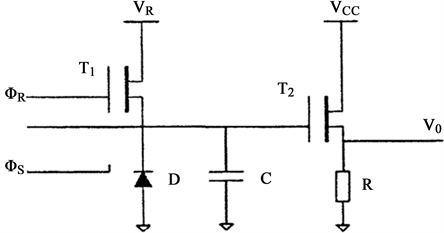Figure 3. Output structure diagram of floating diffusion amplifier

$\stackrel{¯}{{V}_{n}^{2}}=4kTR\Delta {f}_{e}=kT/C$ (58)Figure 4. Output noise equivalent circuit

$\Delta n=\sqrt{kTC}/e$ (59)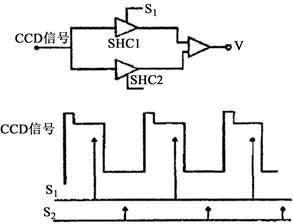Figure 5. Principle circuit and timing waveform of correlated double sampling

b) 低频噪声(1/f噪声)

${S}_{I}\left(f\right)\propto {I}^{\beta }$ (60)

5. 结果与讨论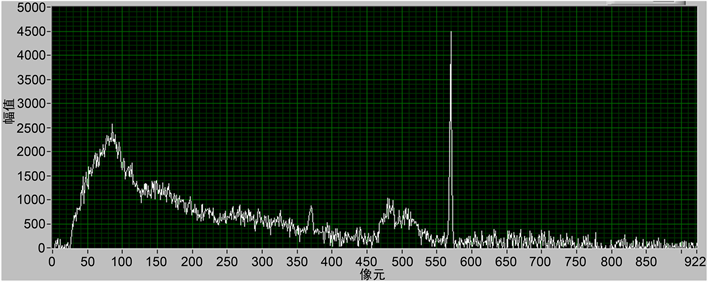Figure 6. Image and gray scale curve with 100 ms exposure time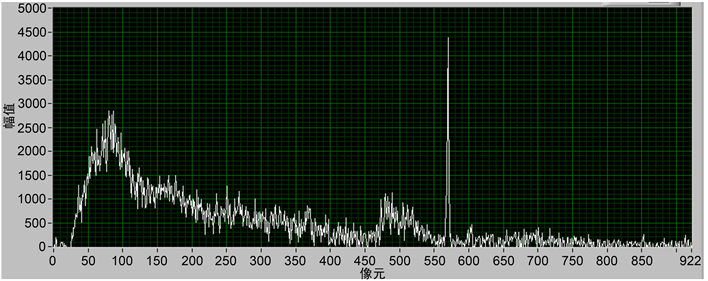Figure 7. Image and gray scale curve with 10 ms exposure time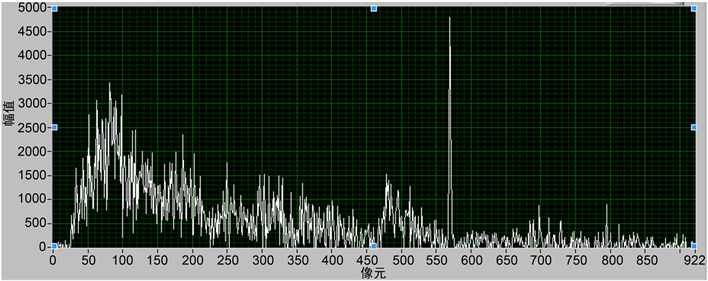Figure 8. Image and gray scale curve with 1 ms exposure time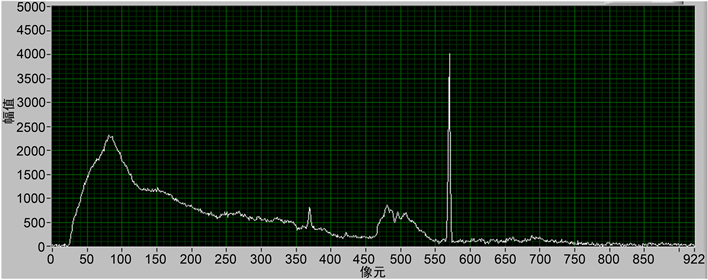Figure 9. The result of smoothing the gray-scale curve with 100 ms exposure time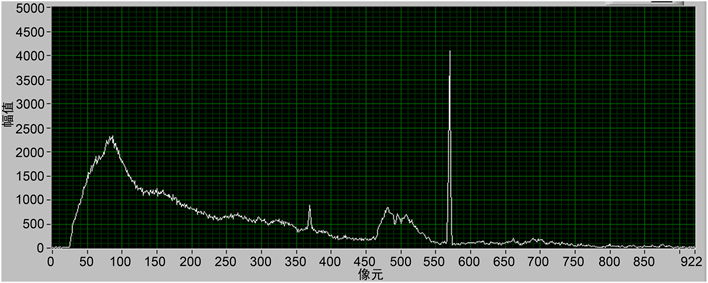Figure 10. The result of smoothing the gray-scale curve with 10 ms exposure time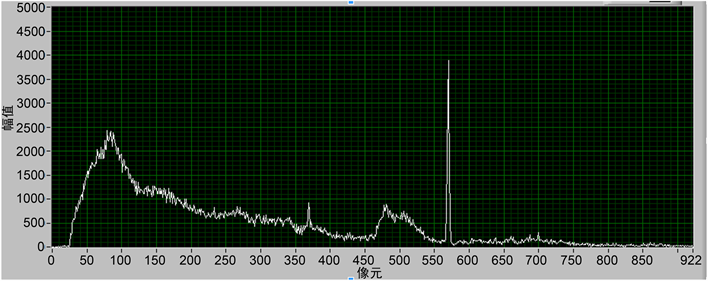Figure 11. The result of smoothing the gray-scale curve with 1 ms exposure time

6. 结论

NOTES

*通讯作者。

  余金中. 半导体光电子技术[M]. 北京: 化学工业出版社, 2003.  李志鹏, 李大成, 王燕燕. 一种基于日盲紫外定焦成像系统的海上搜寻定向系统[P]. 中国专利, CN201822186311.8, 2019-9-6.  Lavigne, C., Roblin, A. and Langlois, S. (2002) Solar-Blind UV Imaging Photon Detector with Automatic Gain Control. Measurement Science & Technology, 13, 713. https://doi.org/10.1088/0957-0233/13/5/309  Ying, L. and Zhu, T. (2008) A Study on Solar Blind UV ICCD Detection Performance. Proc of SPIE, 6621, 21-27  Denvir, D.J. and Conroy, E. (2003) Electron-Multiplying CCD: The New ICCD. International Symposium on Optical Science and Technology, Vol. 4796, Seattle, 3-8 August 2003, 167-174. https://doi.org/10.1117/12.457779  周蓓蓓. 电子倍增 CCD 的工作模式及其光子计数成像研究[D]: [博士学位论文]. 南京: 南京理工大学, 2010. http://dx.chinadoi.cn/10.7666/d.y1919664  Chen, G.P., Gao, Z.S., Zhu, P.J. and Chen, Z.J. (2020) Learning a Mul-ti-Scale Deep Residual Network of Dilated-Convolution for Image Denoising. 2020 IEEE 5th International Conference on Cloud Computing and Big Data Analytics, Chengdu, 10-13 April 2020, 348-353. https://doi.org/10.1109/ICCCBDA49378.2020.9095754  Kumwilaisak, W., Piriyatharawet, T., Lasang, P. and Thatphithakkul, N. (2020) Image Denoising with Deep Convolutional Neural and Multi-directional Long Short-Term Memory Networks under Poisson Noise Environments. IEEE Access, 8, 86998-87010. https://doi.org/10.1109/ACCESS.2020.2991988  Saeedzarandi, M., Nezamabadi-Pour, H. and Jamalizadeh, A. (2020) Dual-Tree Complex Wavelet Coefficient Magnitude Modeling Using Scale Mixtures of Rayleigh Distribution for Image Denoising. Circuits, Systems, and Signal Processing, 39, 2968-2993. https://doi.org/10.1007/s00034-019-01291-y  陈梦雅, 李润鑫, 刘辉, 尚振宏. 基于预滤波的组稀疏残差约束图像去噪模型[J]. 传感器与微系统, 2020, 39(2): 48-51. http://dx.chinadoi.cn/10.13873/J.1000-9787(2020)02-0048-04  常圆圆, 张选德. 基于多尺度相似先验的非局部图像去噪算法[J]. 电脑知识与技术, 2020, 16(2): 200-203.  Seo, S. (2020) Image Denoising and Refinement Based on an Iteratively Reweighted Least Squares Filter. KSCE Journal of Civil Engineering, 24, 943-953. https://doi.org/10.1007/s12205-020-2103-x  闫丰, 于子江, 于晓, 娄洪伟, 隋永新, 杨怀江. 电晕探测紫外ICCD相机图像噪声分析与处理[J]. 光学精密工程, 2006, 14(4): 709-713.  但唐仁, 田景全, 高延军, 等. 低强度X射线影像系统的噪声分析及图像去噪处理[J]. 发光学报, 2002, 23(6): 615-618.  许宏涛, 邵晓鹏, 王杨. CCD芯片性能参数测量系统[J]. 仪器仪表学报, 2011, 32(6增): 271-275.  罗转翼, 程桂芬. 随机信号处理与控制基础[M]. 北京: 化学工业出版社, 2002: 21-22.  刘一畅, 马伟, 徐士彪, 张晓鹏. 基于卷积神经网络的边缘保真图像去噪算法[J]. 计算机辅助设计与图形学学报, 2020(11): 1822-1831.